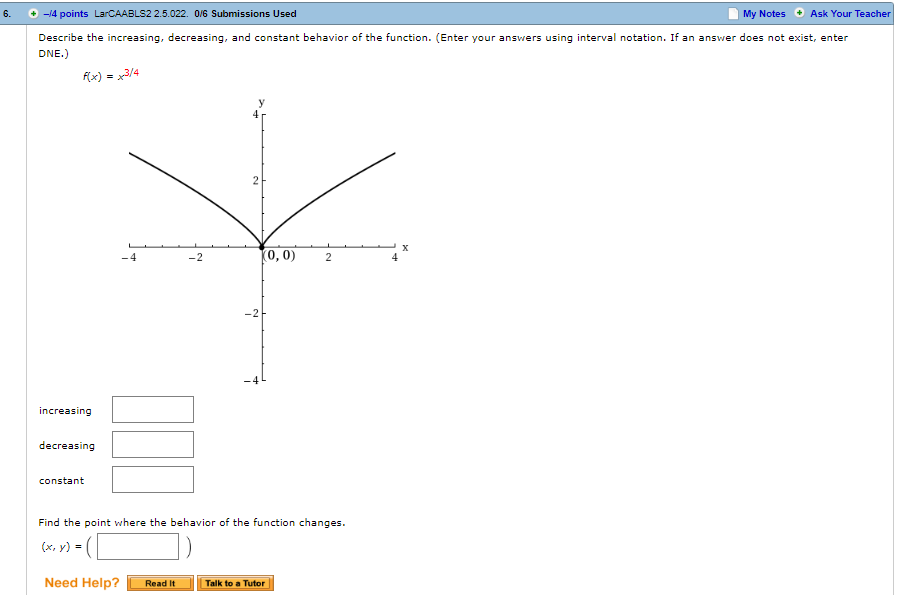# 6. +-4 points LarCAABLS2 2.5.022. 0/6 Submissions Used My Notes Ask Your Te Describe the increasing, decreasing, and constant behavior of the function. (Enter your answers using interval notation. If an answer does not exist, enter 0,0) 2 increasing decreasing Find the point where the behavior of the function changes (x, y)s Need Help?Read It Talk to a Tutor

Question

Describe the increasing, decreasing, and constant behavior of the function. (Enter your answers using interval notation. If an answer does not exist, enter DNE.)help_outlineImage Transcriptionclose6. +-4 points LarCAABLS2 2.5.022. 0/6 Submissions Used My Notes Ask Your Te Describe the increasing, decreasing, and constant behavior of the function. (Enter your answers using interval notation. If an answer does not exist, enter 0,0) 2 increasing decreasing Find the point where the behavior of the function changes (x, y)s Need Help?Read It Talk to a Tutor fullscreen